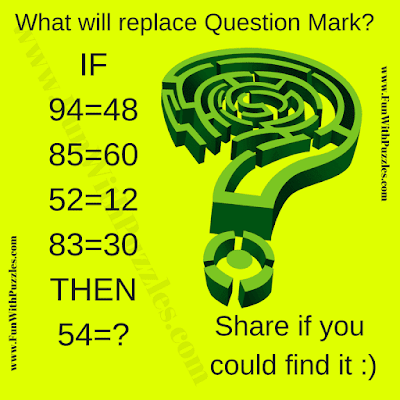## Tuesday, May 22, 2018

Here is Mind-cracking Maths Puzzle for Adults. This is one of the very tough Maths picture puzzle. It will take lot of thinking and Mathematical calculations to solve this Maths Puzzles. Here in this puzzle there are some Mathematical equations and your challenge is to find logical pattern in these equations to solve the last equation and find the missing number which replaces question mark?Can you solve this mind-cracking Maths Puzzle?

Answer of this "Mind-cracking Maths Puzzle", can be viewed by clicking on button. Please do give your best try before looking at the answer.

1.hi, what's the solution to ths riddle? https://www.funwithpuzzles.com/2018/05/mind-cracking-maths-puzzle-for-adults.html

1.Thanks for asking the solution of this Mind Cracking Puzzle. Answer button for this puzzle is enabled now to show the solution of this puzzle.
Do let us know if something is not clear?

2.1.3.1.